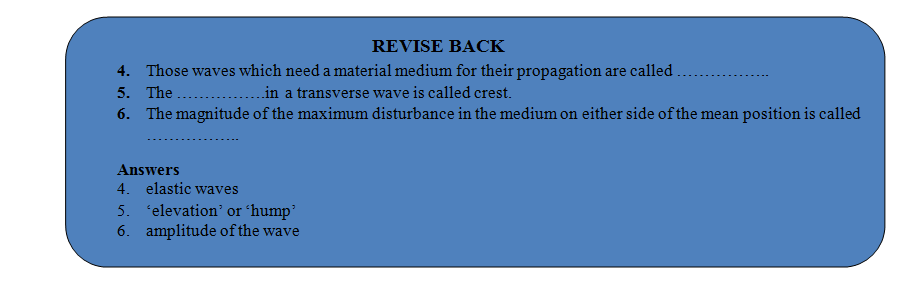# SOUND NEEDS A MATERIAL MEDIUM FOR ITS PROPAGATION

## Sound of Class 9

An electric bell is enclosed inside an inverted bell jar by hanging from the rubber cork. The jar is closed at the bottom by an airtight place with a hole in the centre. A pipe through the hole leads out to a vacuum pump (pump which draw the air out a vessel).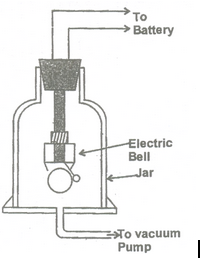The bell is connected to a battery through a key.

The bell is started by closing the key. Initially when jar has normal air inside it, sound waves produced by the ringing bell heard outside the jar.

The vacuum pump is started and the air from inside the jar is gradually drawn out. With decreases air inside the jar, sound heard becomes weaker and weaker. After sometime no sound is heard, though the bell hammer is seen in vibration.

### Conclusion:

In the absence of medium (air) around the source, sound is not being propagated.

A natural fact: Moon has no atmosphere. The space above the atmosphere is also vacuum. If some explosion takes place on moon, sound of the explosion will not be propagated to the earth. So the sound waves never reach the earth.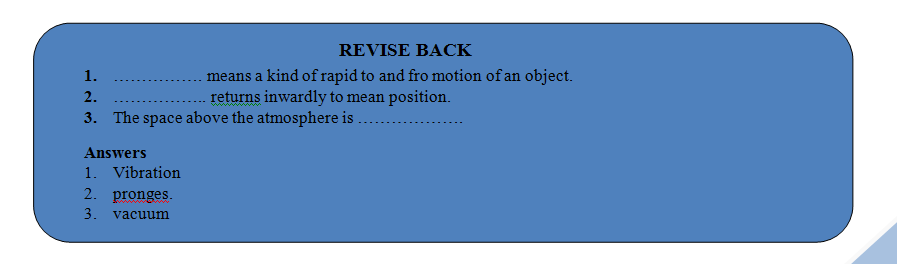### WAVE NATURE OF SOUND:

A wave is a vibratory disturbance in a medium which carries energy from one point to another, without there being a direct contact between the two points.

(a) Type of waves on the basis of material medium

• Elastic waves or mechanical waves: Those waves which need a material medium for their propagation are called elastic waves or mechanical waves e.g., sound waves and water waves are elastic or mechanical waves.
• Electromagnetic waves: Those waves which do not need a material medium for their propagation are called electromagnetic waves. These waves can travel through vacuum as well as through medium e.g. light waves and radio waves are electromagnetic waves.
• Seismic waves :The disturbances inside the earth which cause waves moving in all direction are called seismic waves. It is these waves which cause earth quakes.

(b) Types of waves on the basis of its direction of propagation

• Transverse waves : The waves in which the particles of the medium vibrate up and down ‘at right angles’ to the direction in which the wave is moving, are called transverse waves. Eg., light waves, radio waves, water waves etc.

The water waves (or ripples) formed on the surface of water in a pond are transverse waves. This is because of the fact that in a water wave, the molecules of water move up and down in the vertical direction when the wave travels in the horizontal direction along the water surface. When a stone is dropped in a pond of water, transverse water waves are produced on the surface of water.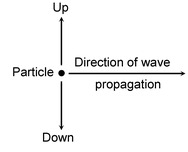The ‘elevation’ or ‘hump’ in a transverse wave is called crest. In other words, a crest is that part of the transverse wave which is above the line of zero disturbance of the medium. In figure, XY is the line of zero disturbance and A and C are the two crests of the transverse water waves.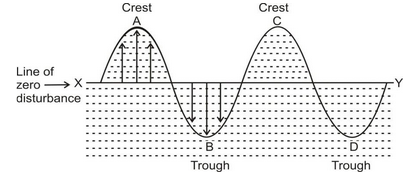The ‘depression’ or ‘hollow’ in a transverse wave is called trough. In other words, a trough is that part of the transverse wave (B and D) which is below the line of zero disturbance.

Longitudinal waves: A wave in which the particles of the medium vibrate back and forth in the ‘same direction’ in which the wave is moving, is called longitudinal wave. Eg. – Sound waves.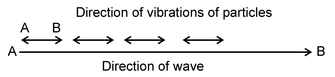Wave motion is a form of disturbance (a mode of energy transfer) which is due to repeated vibrations of the particles of the medium about their mean positions and the motion is handed over from one particle to the other without any net transport of the medium.

### Sound as a Longitudinal Wave:

Sound produced by the object from vibration is known as mechanical wave. The vibrations produced the sound of the object becausethe particles in the surrounding medium transport  vibrational motion, thus transporting energy through the medium then sound produced. For a sound wave traveling through any medium like air, the vibrations of the particles are best described as longitudinal waves. Longitudinal waves are waves in which the motion of the individual particles of the medium is in a direction that is parallel to the direction of energy transport by the waves. A longitudinal wave can be created in a slinky if the slinky is stretched out in a horizontal direction and the first coils of the slinky are vibrated horizontally. Each individual coil of the medium is set into vibrational motion in directions parallel to the direction that the energy is transported.

### Production of compressions and rarefactions near a source of sound:

Air is best example because air is the most common medium through which sound travels and it does so easily with the help of intervening layers of air. A source of sound puts the particles of the medium into vibratory motion. We can understand this by the experiment - Let us consider a vibrating tuning fork as a source of sound.

(a) When the right prong moves from left (L) to right (R), it compresses the layer of air in front of it. This results in the increase of the pressure as well as density of this layer. This layer (or region) of compressed air is called a compression.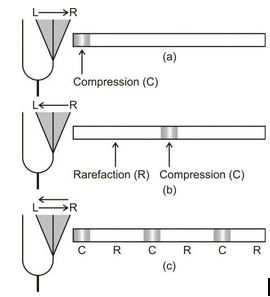(b) When the prong moves from its right extreme (R) to left extreme (L), the air in front of it expands. As a result, the pressure (as well as density) of this layer decreases. This region of rarefied air is called a rarefaction.

Thus,

• A sound wave which propagates as a series of compressions and rarefactions is a longitudinal wave.
• A sound wave can be considered as propagation of pressure or density variations in the medium.

### Characteristics of a Sound Wave:

As already discussed, sound waves are produced due to variations in pressure and density of the medium. The various other characteristics are:

Compression and rarefaction

A compression and rarefaction is formed when the particles of the medium lie farther apart than the normal distance.

• Compression: A portion of the medium where a temporary decrease in volume and consequently a increase in density takes place when a sound wave passes through the medium, is called a compression or a condensation.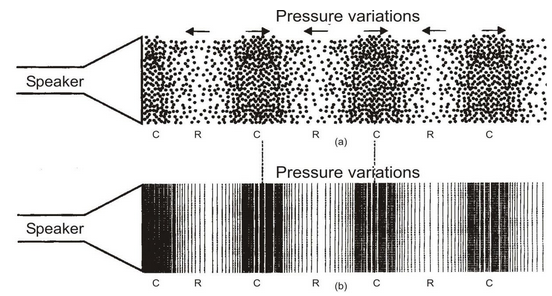• Rarefaction: A portion of the medium where a temporary increase in volume and consequently a decrease in density takes place when a sound wave passes through the medium is called a rarefaction.

Graphical representation of sound wave

The graphical representation of sound wave is given below :

• Crest:The portion of the medium where the density (or pressure) has a value larger than its average value is called a crest.
• Trough : The portion of the medium where the density (or pressure) has a value smaller than the average value is called a trough.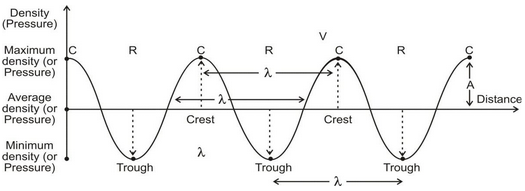The points of maximum density (or pressure) and minimum density (or pressure) are also called crests and troughs respectively.

• Amplitude (A) : The magnitude of the maximum disturbance in the medium on either side of the mean position is called the amplitude of the wave. It is usually represented by the letter A. In case of sound, the unit of A is the same as that of density or pressure.
• Oscillation : As is clear from the graph, the density (or pressure) of the medium oscillates between a maximum value and a minimum value. The change in density (or pressure) from maximum value to the minimum value and again to the maximum value is called an oscillation.
• Frequency (v) : The frequency of a sound wave is defined as the number of complete oscillations per second. It is denoted by the symbol ν (Greek letter, nu).

Unit of frequency is cycle per second (cps) or s1 or hertz (Hz) which is named after Heinrich Hertz (1857-1894).

Bigger units of frequency are kilohertz (kHz, 103Hz) and megahertz (MHz, 106 Hz).

• Time Period (T) : The time taken for one complete oscillation in density (or pressure) of the medium is called the time period of the wave.
• Time period of the wave is also defined as the time taken by its two consecutive compressions or rarefactions to cross a fixed point.
•  Wavelength (λ) : The distance between two consecutive compressions or two consecutive rarefactions is called the wavelength of the wave. It is denoted by the symbol λ (Greek letter, lambda). Wavelength of a sound wave is also equal to the distance travelled by it in its periodic time (T). Unit of wavelength is metre (m).
• Relation between frequency and time period

If the frequency of the wave is ν, then from the definition of frequency,

Time taken for completing ν oscillations = 1 second

And time taken for completing 1 oscillation =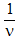second

But time taken for completing 1 oscillation is the period (T) of the wave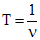or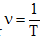or νT = 1 …(1)

• Relation between speed of sound, frequency and wavelength

Speed of sound is the distance travelled by the sound wave per unit time. It is denoted by v and is measured in metre/second (m/s).

From the definition of wavelength,

Distance travelled by sound wave in a periodic time (T) = wavelength (λ) of the sound wave.

∴ speed of sound,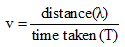i.e.,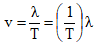Butwhere ν is the frequency of the sound wave.

∴ v = νλ …(1)

If two of the three quantities are known, the third quantity can be obtained from equation (1).

Thus, to describe a sound wave, we need to know :

•  Speed
• Frequency (or wavelength) and
• Amplitude